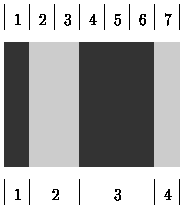Online JudgeProblem SetAuthorsOnline ContestsUser
Web Board
F.A.Qs
Statistical Charts
Problems
Submit Problem
Online Status
Prob.ID:
Register
Authors ranklist
Current Contest
Past Contests
Scheduled Contests
Award Contest
Register
Language:
Bar Codes
 Time Limit: 1000MS Memory Limit: 10000K Total Submissions: 1237 Accepted: 460

Description

A bar-code symbol consists of alternating dark and light bars, starting with a dark bar on the left. Each bar is a number of units wide. Figure 1 shows a bar-code symbol consisting of 4 bars that extend over 1+2+3+1=7 units.Figure 1: Bar-code symbol over 7 units (see top) with 4 bars (see bottom)

In general, the bar code BC(n,k,m) is the set of all symbols with k bars that together extend over exactly n units, each bar being at most m units wide. For instance, the symbol in Figure 1 belongs to BC(7,4,3) but not to BC(7,4,2).
```0: 1000100  |  8: 1100100
1: 1000110  |  9: 1100110
2: 1001000  | 10: 1101000
3: 1001100  | 11: 1101100
4: 1001110  | 12: 1101110
5: 1011000  | 13: 1110010
6: 1011100  | 14: 1110100
7: 1100010  | 15: 1110110
Figure 2: All symbols of BC(7,4,3)```

Figure 2 shows all 16 symbols in BC(7,4,3). Each `1' represents a dark unit, each `0' a light unit. The symbols appear in lexicographic (dictionary) order. The number on the left of the colon (`:') is the rank of the symbol. The symbol in Figure 1 has rank 4 in BC(7,4,3).

Input

Your program is to read from standard input. The first line contains the numbers n, k, and m (1 <= n,k,m <= 33). On the second line is a number s (0 <= s <= 100). The following s lines each contain some symbol in BC(n,k,m), represented by '0's and '1's as in Figure 2.

Output

Your program is to write to standard output. On the first line your program should write the total number of symbols in BC(n,k,m). On each of the s following lines, it should write the rank of the corresponding symbol in the input.

Sample Input

```7 4 3
5
1001110
1110110
1001100
1001110
1000100```

Sample Output

```16
4
15
3
4
0```

Source

[Submit]   [Go Back]   [Status]   [Discuss]Home PageGo BackTo top